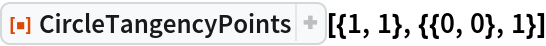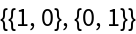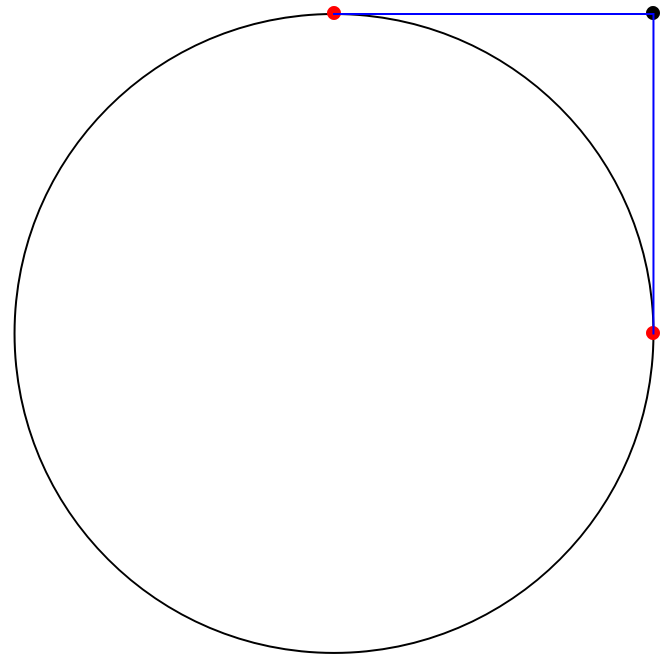Function Repository Resource:

# CircleTangencyPoints

Find the tangency points on a circle emanating from a point

Contributed by: Thomas Shundi
 ResourceFunction["CircleTangencyPoints"][{a,b},{{h,k},r}] returns tangency points {{x1,y1},{x2,y2}} on the circle centered at {h,k} with radius r which also pass through the point {a,b}.

## Details

ResourceFunction["CircleTangencyPoints"] returns an empty list when the given point is inside of the circle, and returns the point itself if it lies on the circumference of the circle.

## Examples

### Basic Examples (2)

Given the point {1,1} and the unit circle centered at the origin, CircleTangencyPoints returns the coordinates of the two tangency points:

 In:=Out=Here is a diagram illustrating this result:## Publisher

Wolfram Summer Camp

## Version History

• 1.0.0 – 20 January 2021